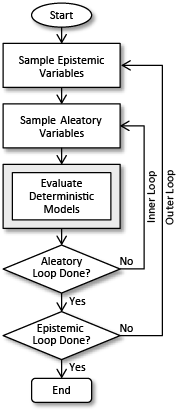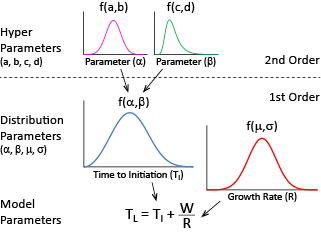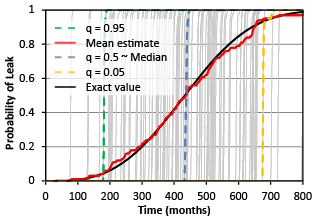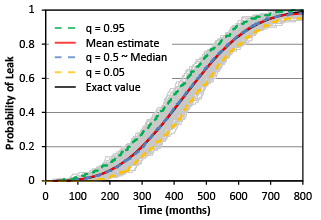# Uncertainty Analysis## Separation of aleatory and epistemic uncertainties in probabilistic assessments

The separation of aleatory and epistemic uncertainties has been a source of interest in many studies.  The uncertainties have been characterized as:

• Aleatory Uncertainty: Referred to as simply variability, or random (or stochastic) heterogeneity in a population that cannot be reduced.
• Epistemic Uncertainty: Refers to any kind of lack of information (i.e., ignorance) with respect to model parameters, variables, structure or form, which may be reduced by further measurement or study.To quantify the contribution of each uncertainty on the final results, the uncertainties are typically separated in a two-staged nested Monte Carlo simulation approach, where the epistemic parameters are sampled in the outer loop, while the aleatory variables are simulated as part of the inner loop.

However, the process of uncertainty separation may lead to misinterpretation of the results, particularly with respect to the confidence (i.e., lower and upper bounds) in the estimated probabilities.  Much of the confusion is related not only to how the uncertainty in the variables and parameters is defined (i.e., which uncertain input variables should be described as aleatory as opposed to epistemic), but also the way in which the uncertainties are propagated to the final results.

Naturally, the results are also directly impacted by any uncertainties in the underlying model itself (i.e., the ability and applicability of  mathematical equations to represent "reality").  This model uncertainty is a fundamental problem with all computational models, and is exceedingly difficult to quantify.

### Order in uncertaintyThe two-staged Monte Carlo simulation approach is directly applicable to second-order random variable problems, where the parameters of the uncertain input variable distributions are themselves considered to be random variables.

The basic (first-order) uncertain input variables are sampled in the inner loop (i.e., considered as "aleatory" variables), while the (second-order) uncertain parameters would be sampled in the outer (epistemic) loop.

This approach leads to consistent results, that reflect the relative contribution of each type of uncertainty in the final estimates.

The main difficulty occurs when the (first-order) uncertain input variables (and/or any second-order parameters) are arbitrarily characterized as either aleatory or epistemic.  Many models, including the xLPR project on probabilistic fracture mechanics, allow many of the model parameters and variables to be designated either as epistemic or aleatory (or also constant).  While beneficial to the end user, the arbitrary separation of variables may lead to unexpected results, specifically with respect to the confidence intervals (i.e., upper and lower bounds) of the estimated probabilities.

### Simple example

Consider the following simple model for the time to leak for a pipe, for example, from stress corrosion cracking, as

T_L = T_I + \frac{W}{R}

where T_L is the uncertain time to leak, T_I is the time to crack initiation (assumed to be a random variable), W is (a constant) wall thickness of the pipe, and R is the crack growth rate (also assumed to be a random variable).  The term W/R represents the time it takes for an initiated crack to grow through the pipe wall, resulting in a leak.Assume the random time to initiation T_I is arbitrarily assumed to be epistemic (and hence simulated in the outer loop), while the random growth rate R is inherently random (i.e., aleatory, and hence sampled in the inner loop).

The adjacent figure illustrates the estimated distribution of probability of leak over time, including the lower and upper bounds (with q corresponding to the percentile level), for 100 inner and 100 outer simulation trials.  Each grey line in the figure is commonly referred to as a “hair”, and represents a single realization (out of 100) of the time to leak distribution for each outer (epistemic) loop in the simulation.Compare this result to the opposite case in the figure on the right, where the random growth rate R is now assumed to be epistemic (and hence, sampled as part of the outer loop), while the random time to initiation T_I is assumed to be inherently random (and thus part of the inner aleatory loop).  As before, both loops utilized 100 simulation trials.

While the mean estimates are the same in both cases, it is evident that the estimated lower and upper bounds are clearly very different.  The difference is due to the fact that the bounds are "conditional" on the arbitrarily separated variables.

The bounds reflect the variation of all variables sampled in the outer (epistemic) loop (corresponding to the time to initiation T_I in the first case, and the growth rate R in the second case).  The bounds, therefore, reflect the "sensitivity" of the model output to each of the separated variables, which is useful in the context of sensitivity analysis.  As a result, the true confidence bounds of the underlying output variable (i.e., probability of leak) cannot be determined in this context (only the mean estimate is possible, as the conditional expectations are the same).

### Summary and conclusions

The two-staged nested Monte Carlo simulation approach is most applicable to second-order random variable problems.  For first-order problems, the

• Probability of leak (or rupture) is a fixed number
• Uncertainty bounds on the probability are not dependent on the uncertainty in the input variables, but only on the uncertainty arising from estimation (i.e., simulation sample size)
• Separation of variables leads to sensitivity bounds, which reflect the uncertainty in the input variables (designated as epistemic in the outer loop), not the probability itself

For second-order problems, the

• Probability of leak (or rupture) is a random variable
• Uncertainty bounds reflect both second-order parameter (epistemic) uncertainty and the uncertainty from estimation